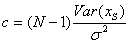# One sample chi-square test

This test is used to check hypotheses about the fact that dispersion of random variable X represented by sample x equals given σ 2.

During its work, the test calculates the c-statistic:If X has a normal distribution, the c-statistic will have a chi-square distribution with N-1 degrees of freedom. To define the significance level which corresponds to the value of c-statistic high-precision chi-square distribution approximation is used. Test returns three p-values:

• p-value for two-tailed test (null hypothesis - the dispersion is equal to the given number)
• p-value for left-tailed test (null hypothesis - the dispersion is greater than or equal to the given number)
• p-value for right-tailed test (null hypothesis - the dispersion is less than or equal to the given number)

# Two-sample F-test

This test checks hypotheses about the fact that the dispersions of two random variables X and Y which are represented by samples xS and yS are equal. The test works correctly under the following conditions:

• both random variables have a normal distribution
• the samples are independent

During its work, the test calculates the F-statistic:If X and Y have a normal distribution, the F-statistic will have F-distribution with NX -1 and NY -1 degrees of freedom. To define the significance level which corresponds to the value of F-statistic high-precision, F-distribution approximation is used.

Test returns three p-values:

• p-value for two-tailed test (null hypothesis - the dispersions are equal)
• p-value for left-tailed test (null hypothesis - the dispersion of the first sample is greater than or equal to the dispersion of the second sample)
• p-value for right-tailed test (null hypothesis - the dispersion of the first sample is less than or equal to the dispersion of the second sample)

ALGLIB Project offers you two editions of ALGLIB:

ALGLIB Free Edition:delivered for freeoffers full set of numerical functionalityextensive algorithmic optimizationsno low level optimizationsnon-commercial license

ALGLIB Commercial Edition:flexible pricingoffers full set of numerical functionalityextensive algorithmic optimizationshigh performance (SMP, SIMD)commercial license with support plan

## ALGLIB 3.15.0 for C++C++ library.
Delivered with sources.
Monolithic design.
Extreme portability.
Editions:   FREE   COMMERCIAL

## ALGLIB 3.15.0 for C#C# library with native kernels.
Delivered with sources.
VB.NET and IronPython wrappers.
Extreme portability.
Editions:   FREE   COMMERCIAL

## ALGLIB 3.15.0 for DelphiDelphi wrapper around C core.
Delivered as precompiled binary.
Compatible with FreePascal.
Editions:   FREE   COMMERCIAL

## ALGLIB 3.15.0 for CPythonCPython wrapper around C core.
Delivered as precompiled binary.
Editions:   FREE   COMMERCIAL